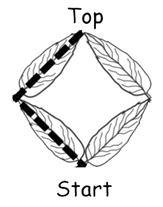Problems
10059 - Ant and the beanstalk

Ant and the beanstalk

Time Limit: 0.5 sec

The Problem

Ant climbed the beanstalk. He always went upwards. He start from bottom and want to reach top. He can do it different ways.

When a beanstalk size is 1 square, he did it like this: left, right. Or he did it like this: right, left.

So, Ant climbed the beanstalk 2 different ways when beanstalk size is 1 square.When a beanstalk size are 4 squares, he did it like this: left, right, left, right. Or he did it like this: left, left, right, right. Or he did it like this: left, right, right, left. And so on.Now, your job is to find how many different way the Ant climbed a beanstalk when the size of beanstalk are n squares.

The Input

The first line contains a positive integer T ( 0<T<1001), denoting the number of test cases. The next T lines will contain one positive perfect square number n (n<901).

The Output

For each test case print "Case X: Y" without quete. Here X denoted the case number and Y denoted the number of different way the Ant climbed to the beanstalk when the size of square is n.

Sample Input

3

1

4

36

Sample Output

Case 1: 2

Case 2: 6

Case 3: 924

[Note: In mathematics, a square number or perfect square is an integer that is the square of an integer; First 6 square numbers are 1, 4, 9, 16, 25, 36]

Online Practice Contest 3 [School & College Level]

Problem Setter: Shahin ShamS